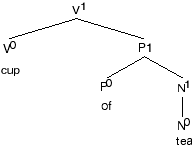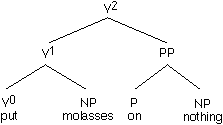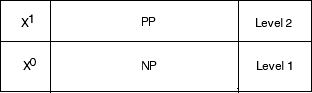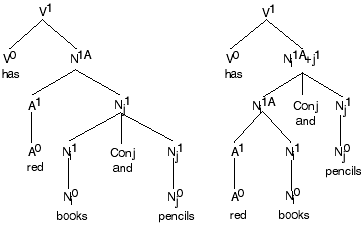Bottom Up Rules

322

X1

Phrase structure rules should be written from the bottom up. By this, it is meant that a head category is selected, and the structure is built up from the head. For example, let's start with 'V', which is the starting point for most sentences. Initially we could say that V and is complement, which is a NP, projects to VP:

V (NP) <--> VP. The rules states that V and its complement if the verb takes one is projected to VP. Reading from right to left we could say the VP contains a verb and NP if the verb takes one.

However, there are intermediate levels. First, let's write the lowest level of V as 'V0'. '0' always means the head level.

This specific rules is derived from the more general rules for the expansion of heads to the first level up, which is identified by the superindex 'n+1':

X0 (Comp-X) <--> X1.

This rule states that a head and its complement if it has one is projected to X1. This the only case where X0 must be projected to X1. Examples, one of each type. A projection includes what a head is projected up to. X0 - X1 is called the projection of X. X represents one of the four currently assumed categories. The NP sister of V1is traditionally called the direct object of the verb. The term direct object is not extended to the complements of the remaining heads. The NP object of P is sometimes called a prepositional object:

[V1 eat bananas] or [V1 [V0 eat] [N1 [N0 bananas]]]

[N1 cup of tea] or [N1 [N0 cup] [P1 [P0 of] [N1 [N0 tea]]]]

[A1 fond of syntax] or [A1 [A0 fond] [P0 of] [N1 [N0 syntax]]]]

[P1 on the shelf] or [P1 [P0on] [NP the shelf]]X2

The next projection creates X2. This level includes a PP- complement, V0 - V1 - V2 is the projection of V:

Jason put molasses on nothing.

X1 (Comp2-X) <--> X2.Molasses is the direct object ’Äî a level 1 complement simply called Comp-X (Comp-V, here). Level 2 complements are always PPs. We label then Comp2-X. The evidence for binary branching is discussed in the 222 notes; it is based the fact that adverbs may occur between Comp-X and Comp2-X. Comp-X is a sister of X0, and Comp2-X is a sister of X1:

Jason put molasses deliberately on the chair.

In the 222 notes you will note that the adverb must be adjoined to V1. Other examples of expanding the X2 rule are given below. P may take a Comp2 complement but comp2 and comp1 complements do not appear to occur at the same time. I can't think of any adjectives that take both:

[V2 [V1places the papers] on the desk]

[P2 [P1 from] [ P2 [P1 under the bed]]]

[N2 [P1 gift of flowers] to Molly]

P2 in tree structure form is the following:

X2a, b, c

Next, there may be more than one complement at level 2. In this case, the first PP is written as PPa, the second one as PPb, and so forth for each complement starting from the left:

Mary pushed the pram from the pool through the garden to the bench.

The pushing of the pram from the pool through the garden to the bench.

There are no known equivalent examples for P and A. The rule expansion automatically assigns he superscript 'a' to the first complement. When there is only one complement, the 'a' is usually not written. The rule expansion:

X1 (Comp2-X) <--> X2a.

X2a Comp2-X <--> X2n+1. (The rule applies from left to right. 'n' here represents an alpha-numeric symbol starting with 'a', the next one (n+1) will be 'b', and so forth. Note that Comp2 is mandatory for the expansion to occur. If there is no further complements or no C0mp2 complements at all, then proceed to the next expansion (Xc).

The Comp-X and Comp2-X thing is not complicated. If the complement is an NP, it is assigned to level-1, the sister level to X0. If it is a PP, it is assigned to level-2, the sister level to X1. You can think of it as:The first level complements of A and N are 'of NP'. This not really an exception to the above. 'Of' here is a functional preposition. The story of 'of' will be covered later in a later chapter (the theory of abstract Case). The above figure is temporarily the following:

The next expansion is to XCH which will be covered later.

The top level, Xch, is a highest projection and called maximal projection. See the section on projections. It is convenient to write a maximal project as XP. Actually, the maximal projection is the highest level, whether the top level is reached or not. A maximal projection must be a phrase. Therefore, V1 is a maximal projection if V1 cannot be projected to V2.

Lexical Modifiers

Lexical modifiers are adjoined to XP, as we now know it. Recall XP is a phrase, and it can be X1 or X2 or even XCH, whatever the highest expansion in the projection is.

The red and white book.

Here, both adjectives c-command book. C-command is required for modification. In the simple form, we can let XP immediately dominate XP. In the more explicit and technically accurate form, start with XPA and apply the now somewhat familiar rule:

XPA <--> AP XPB or by formula:

XPA <--> AP XPA+1, where 'n' is alphanumeric starting with A=1, B=2, and so forth.

By convention the projection with modifiers will be marked with a capitol letter, A, B, and so forth. The small letter 'a' will be reserved for the three or level 2 PPs.

The determiner the is omitted in the following diagram:

The following sentence is ambiguous:

Jason has a red books and pencils.

It means either that Jason has red books and red pencils, or it means that Jason has pencils and red books. The ambiguity is resolved by whether the adjective is adjoined to books only or whether it is adjoined to books and pencils:In the first structure red c-commands both books and pencils modifying both, whereas in the second structure red c-commands books only, thus modifying books but not pencils. When nodes are conjoined, '+' is used to indicate the conjunction of two categories, where '+' connects the subindices. Normally, NP would suffice. I will have you do it this way now and the we can use the NP form when you have it under your belt.

The modifier must be adjoined the maximal projection of N (for now, anyway). 'very hot cup of green tea' has the following structure:

[Note: Get rid of "A" in 'very']

Here, every head is given a subindex except for the functional preposition 'of.' The reason it doesn't get one is because it is not lexical and has no reference in this or any other world.

There are too many parts of speech called adverbs. I will introduce the simplified system of adverbs:

Here, an adverb is a lexical word which modifies a verb or an adjective:

slowly tear up the paper

rapidly drive a car

carefully complete an exercise

John died slowly.

*Mary killed quickly a mosquito.

Eloise spoke excitedly to her T.A.

Adverbs may occur after the lowest verb phrase. If the adverb does not end in the suffix '-ly', it may not occur before the verb:

That student runs quickly.

A few foxes scamper fast.

*A few foxes fast scamper.

The rule:

The adverb rule will have to be modified later.

However, it does not stop here. Adverbs can be adjoined to the right of any phrasal level of the verb:

John read a book slowly to his kids. (to V1).

John read a book to his kids slowly. (to V2).

Mary rode her bicycle slowly from school to the store.

Mary rode her bicycle from school slowly to the store.

Mary rode her bicycle from school to the store slowly.

The position of these adverbs is part of the argumenttion for the various levels of Xn: X1 + Adv + X2a + Adv + X2b+ Adv + X2c.

Operators:

Operators are modifiers that are required by the grammar. Operators cannot be deleted. An empty operator usually has a minus value. The first operator that is always discussed in text books is the node D for [+Definite] also called determiners. If D is not definite, it has the feature [-Def]. Operators are distinct from lexical modifiers in one important way in addition to being obligatory in the grammar. If an operator position is phonetically null, it is assigned the minus value, which stands in opposition to the positive value. For example, 'the rain' is [+Def], and 'rain' is [-Def]. The meaning of [+Def] does not overlap with the meaning of [-Def]:

The circle represents the set Definite, and the upper half is for [+Def] and the bottom for [-Def], which is phonetically null. There is no overlap.

If a lexical operator is null, the opposite meaning is not implied. The information is simply not given:

The red book

The book

In the second sentence, the colour of the book is not implied in any way. Pragmatic knowledge may tell us that books have colour, but which colour is not available here.

The entire circle is the set colour. One of the members of the set is red. If a particular colour is not selected as a member, then no particular colour is selected and colour is not implied. This information is simply missing.

Operators are adjoined to the maximal projection of X including modifiers. Since operators are obligatory for the class they modify (have scope over), the new maximal projection must dominate all operators of that projection.

Determiners

As far as I have been able to determine, specifiers have never been functionally defined. A specifier tends to be defined as the sister of ’âà (X-bar), immediately dominated by XP. The specifier of a noun is a determiner. It appears that specifiers are equivalent to operators. We will rework ’âà Theory such that specifiers are operators. This means the concept of ’âà Theory and specifiers will be modified.

’âà is the level at which operators are adjoined to the projection of X. Following the convention we have been establishing here, the first level is ’âà0. The following noun phrase:

the dog

has the following structure (at this point):

There are two more determiners: this and these, and that and those. The former marks closeness or proximity to the speaker,that latter distance from the speaker. Both terms are relative.

Quantifiers

The second operator is Q for Quantifier. All numerals, whether whole numbers, integers, fractions, decimals, irrational, or imaginary are Q. Q can become complicated, but we discuss only the simple case here. Operators are thought to be closed categories. There are only three pure determiners. Aren't there an infinite number of numbers (quantifiers)? The answer is yes, but this is not the entire story. Every number can be predicted by following a set of rules. In some sense, the numbers are there, it is a matter of mathematical rules that produces them. There is a convention for naming numerals, at least until they get very high or very abstract. There is no pattern for creating new lexical items. They can be created on the spot, and it is a matter of chance whether each one will be accepted into the language, such as words like gazillion, meaning adding unspecified number of zeros to million. Quantification is required in English, but the rules are a bit complex.

The third operator which includes {all, both, each, every, any} we will discuss later. We will assume that operators are specifiers in the standard jargon.

There is one more operator for nouns, the negative operator 'not'. I am not going to include the negative operator now or any other operators that there may be.

Operators are adjoined to a maximal project in ascending order. Since most students are acquainted with D, let us start there. Considering X1 or X2 to be a maximal projection is not quite in sync with that standard theory. D is adjoined to XP forming a higher maximal projection. We will use the now familiar superscript '1' to mark each level. For example, as the structure for 'the three dogs' is given:

[¬µ2 = NP0 [D0 the] [¬µ1 [Q0 three] [N1= ¬µ0 [N0 dogs]]]]].

In tree form:

Currently, it is not necessary to write NP0. Later, it become necessary.

Operators are obligatory for the category they modify. For example, the dog has the following structure. Phonetically null operators and lexical heads are written as "[null]":

Operators are strictly ordered in many languages. In English D must precede Q:

QP ¬µ0 <--> ¬µ1

DP ¬µ1 <--> ¬µ 2

¬µmax = NP

N+modifiers max = ¬µ

the three dogs

*three the dogs

In review the ’âà schema is the following:

XP can be used as a shorthand when the intermediate operators are not indicated. There could be more operators, but they are uncertain at this time, but obviously it will follow the formula ’âàn+1. These dominate operators = specifiers. The operator/specifiers of non-nouns will be covered later.

’âà = maximal phrase where operators are adjoined. ’âà dominates all complements and modifiers.

X+modifiersmax = ’âà.

’âàmax = XP

The labelling convention in review:

The following are not phrase structure rules; the indicate the method of labelling each categories in each component:

X0 - X1 - X2 = the projection of complements.

X2a - X2b - X2c - ... - X2n = the sublevels of PP complements which represent complements of verbs of motion and time and any verb that denotes both a goal and source. The alphanumeric symbol represents linear order from the head.

XnA - XnB - XnC - ... - XnN = the levels of modifiers. the capital alphanumeric symbol represents linear order from the head in both directions. So far we have seen only adjectives, adverbs, and degree words.

X-bar0 - X-bar1 - X-bar2 - ... X-bar3.

The phrase structure rules follow a different convention:

X0 (Comp-X) <--> X1 (Obligatory)

Xn Comp-X <--> Xn+1 (This rule applies if there is a complement), where 'n' ’â€ 1.

X1A\Mod-X <--> Xn+1 where 'n' is alphanumeric in caps, X\Mod means Mod can occur on eather sideof X. In English, simple AP (no complement) occurs to the left of N, PPs to the right of N, AP to either side of Vn, and PPs to the right of Vn. Mod=Degree Word to the left of X.

This is enough for now. There are PP adjuncts and relative adjuncts. We will discuss these later.Course Outline